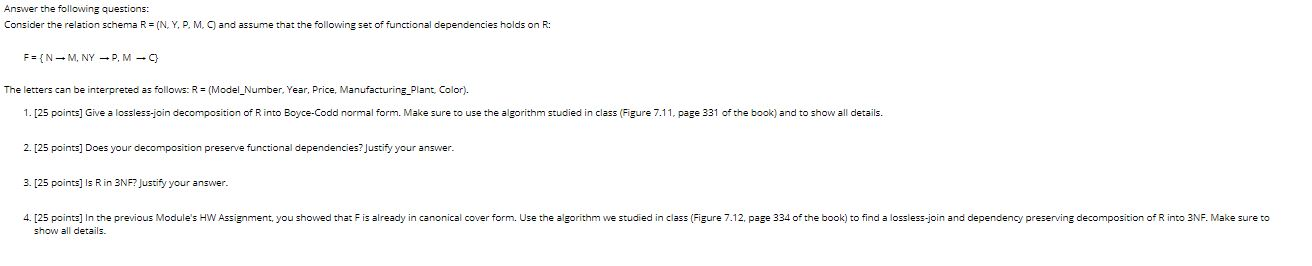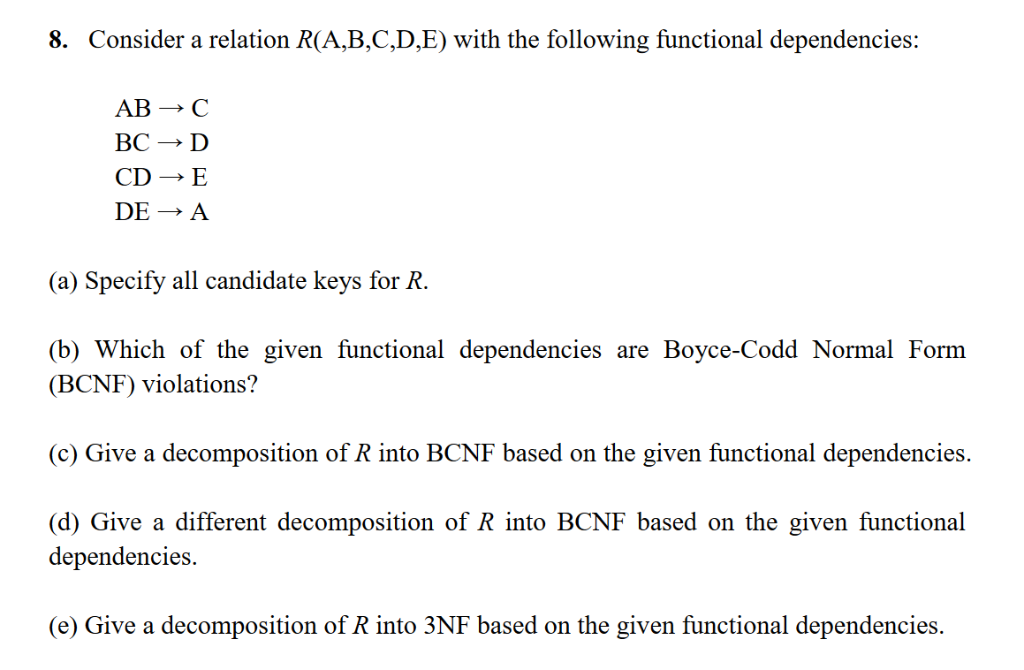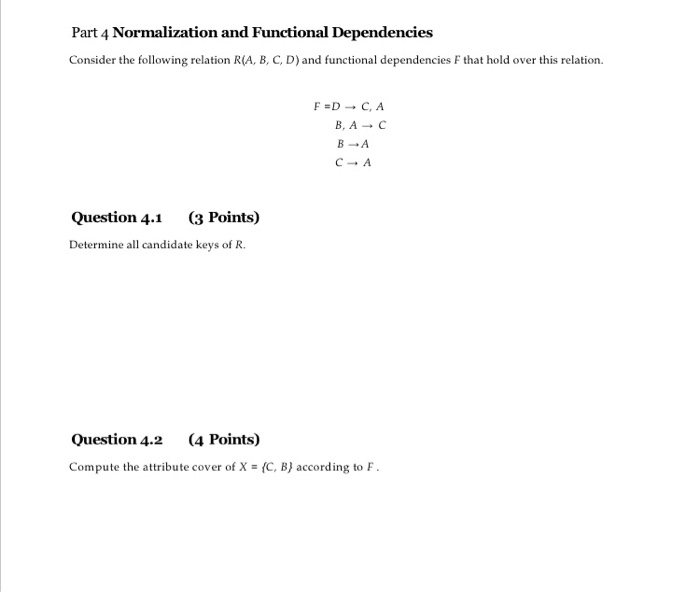Homework Help Question & Answers

# Answer the following questions: Consider the relation schema R = (N. Y, P. M. and assume...Answer the following questions:

Consider the relation schema R = (N. Y, P. M. and assume that the following set of functional dependencies holds on R:

The letters can be interpreted as follows: R=(Model_Number. Year, Price, Manufacturing Plant Color).

1. [25 points] Give a lossless.join decomposition of Rinto Boyce-Codd normal form. Make sure to use the algorithm studied in class (Figure 7.11, page 331 of the book) and to show all details. 2. [25 points] Does your decomposition preserve functional dependencies? Justify your answer.

3. [25 points] Is Rin 3NF? Justify your answer.

4. [25 points] In the previous Module's HW Assignment, you showed that Fis already in canonical cover form. Use the algorithm we studied in class (Figure 7.12, page 334 of the book) to find a lossless-join and dependency preserving decomposition of Rinto 3NF. Make sure to show all details

#### Homework Answers

Answer #1 ✔ Recommended Answer

The relation given is R ( N, Y, P, M, C ) .

The set of functional dependencies given is :

N -> M

NY -> P

M -> C

The candidate key of the given relation is NY because the attribute closure of NY is {N, Y, M, P, C}.

1.

A relation is said to be in BCNF if the left side attribute of every functional dependency is a superkey. Every candidate key is also a superkey.

The following points are considered for a lossless join decomposition of R into Boyce-Codd normal form :

• Include all the attributes in table T1.
• The first functional dependency is N -> M and this violates the BCNF definition because the left side attribute N is not a superkey. So, a separate table T2 has to be created for the attributes N and M with N being common to both T1 and T2.
• The second functional dependency is NY -> P and this follows the BCNF decomposition. So, no separate tables are created.
• The third functional dependency is M -> C and this violates the BCNF definition because the left side attribute M is not a superkey. So, a separate table T3 has to be created for the attributes M and C with M being common to both T1 and T3.

So, the tables formed as a result of lossless join decomposition of R into Boyce-Codd normal form are :

• T1 ( N, Y, P ) with NY as the primary key.
• T2 ( N, M ) with N as the primary key.
• T3 ( M, C ) with M as the primary key.

2.

In T1, the set of functional dependency F1 is :

NY -> P

In T2, the set of functional dependency F2 is :

N -> M

In T3, the set of functional depedency F3 is :

M -> C

Closure of ( F1 U F2 U F3 ) is same as the closure of the original set of functional dependency.

Hence, the decomposition is depedency preserving.

3.

A relation is in 3NF if either the left side attribute in every functional dependency is a superkey or the right side attribute of the same functional dependency is a prime attribute.

The given relation is not in 3NF because in the functional dependency M -> C, neither attribute M is a superkey nor attribute C is a prime attribute. Moreover, in the functional dependency N -> M, neither attribute N is a superkey nor attribute M is a prime attribute.

Hence, the relation is not in 3NF.

4.

The following points are considered a lossless decomposition of R into 3NF :

• Include all the attributes in table T4.
• The first functional dependency is N -> M and this violates the 3NF definition because neither attribute N is a superkey nor attribute M is a prime attribute. So, a separate table T5 has to be created for the attributes N and M with N being common to both T4 and T5.
• The second functional dependency is NY -> P and this follows the 3NF decomposition. So, no separate tables are created.
• The third functional dependency is M -> C and this violates the 3NF definition because neither attribute M is a superkey nor attribute C is a prime attribute. So, a separate table T6 has to be created for the attributes M and C with M being common to both T5 and T6.

So, the tables formed as a result of lossless join decomposition of R into 3NF are :

• T4 ( N, Y, P ) with NY as the primary key.
• T5 ( N, M ) with N as the primary key.
• T6 ( M, C ) with M as the primary key.
Add a comment
Know the answer?
Your Answer:

#### Post as a guest

Your Name:

What's your source?

#### Earn Coin

Coins can be redeemed for fabulous gifts.

Similar Homework Help Questions
• ### Consider a relation R(A,B,C,D,E) with the following functional dependencies: 8. AB C BCD CDE DEA (a) Specify all candidate keys for R. (b) Which of the given functional dependencies are Boyce-Codd No...Consider a relation R(A,B,C,D,E) with the following functional dependencies: 8. AB C BCD CDE DEA (a) Specify all candidate keys for R. (b) Which of the given functional dependencies are Boyce-Codd Normal Form (BCNF) violations'? (c) Give a decomposition of R into BCNF based on the given functional dependencies. (d) Give a different decomposition of R into BCNF based on the given functional dependencies. (e) Give a decomposition of R into 3NF based on the given functional dependencies. Consider a...

• ### Language: SQL - Normalization and Functional Dependencies Part 4 Normalization and Functional Dependencies Consider the following relation R(A, B, C, D)and functional dependencies F that hold o...Language: SQL - Normalization and Functional Dependencies Part 4 Normalization and Functional Dependencies Consider the following relation R(A, B, C, D)and functional dependencies F that hold over this relation. F=D → C, A B,A-C Question 4.1 (3 Points) Determine all candidate keys of R Question 4.2 (4 Points) Compute the attribute cover of X-(C, B) according to F Question 43 (5 Points) Compute the canonical cover of F.Show each step of the generation according to the algorithm shown in class....

Free Homework App

Scan Your Homework
to Get Instant Free Answers
Need Online Homework Help?

Get Answers For Free
Most questions answered within 3 hours.